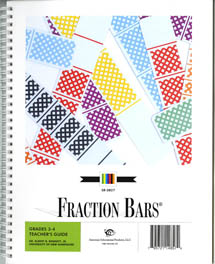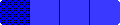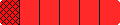Home

Grades 3-4 Fraction Bars Teacher's Guide & Resource Package
Table of Contents IntroductionSpecial Features

Reproducible Materials
Sample Lessons Fraction Bars Games
Teaching Students Fraction Concepts Fraction Bars Materials

Standards for Fractions

Grades 3-4 Fraction Bars Teacher Resource Package (under separate cover)
The Grades 3-4 Fraction Bars Teacher's Guide contains complete teaching information for fractions at these grade levels. There are lessons for the following topics: fraction terminology; equality; inequality; measuring length; relating fractions, decimals, and percents; addition; subtraction; multiplying fractions by fractions; and probability. Games for use with Fraction Bars and Fraction Playing Cards are an integral part of these lessons. At the back of the guide there are 21 black and white masters for use with the lessons. All program components, such as Fraction Bars, Fraction Playing Cards, games, activity mats, and activity sheets are referenced at point-of-use in each step.
Ordering Information for Grades 3-4 Teacher's Guides, Resource Package, and other Grades 3-4 Materials